# Selina Solutions Concise Mathematics Class 6 Chapter 11: Ratio Exercise 11(A)

Selina Solutions Concise Mathematics Class 6 Chapter 11 Exercise 11(A) has problems on Ratios, in its simplest form, along with illustrations. Ratio is the relation between two quantities, obtained on dividing one quantity by the other. Students who find difficulties in solving exercise wise problems, can make use of Selina Solutions PDF to practice textbook questions and also clear their doubts. Selina Solutions Concise Mathematics Class 6 Chapter 11 Exercise 11(A), PDF links are available to know more about the topics, with free download option

## Selina Solutions Concise Mathematics Class 6 Chapter 11: Ratio Exercise 11(A) Download PDF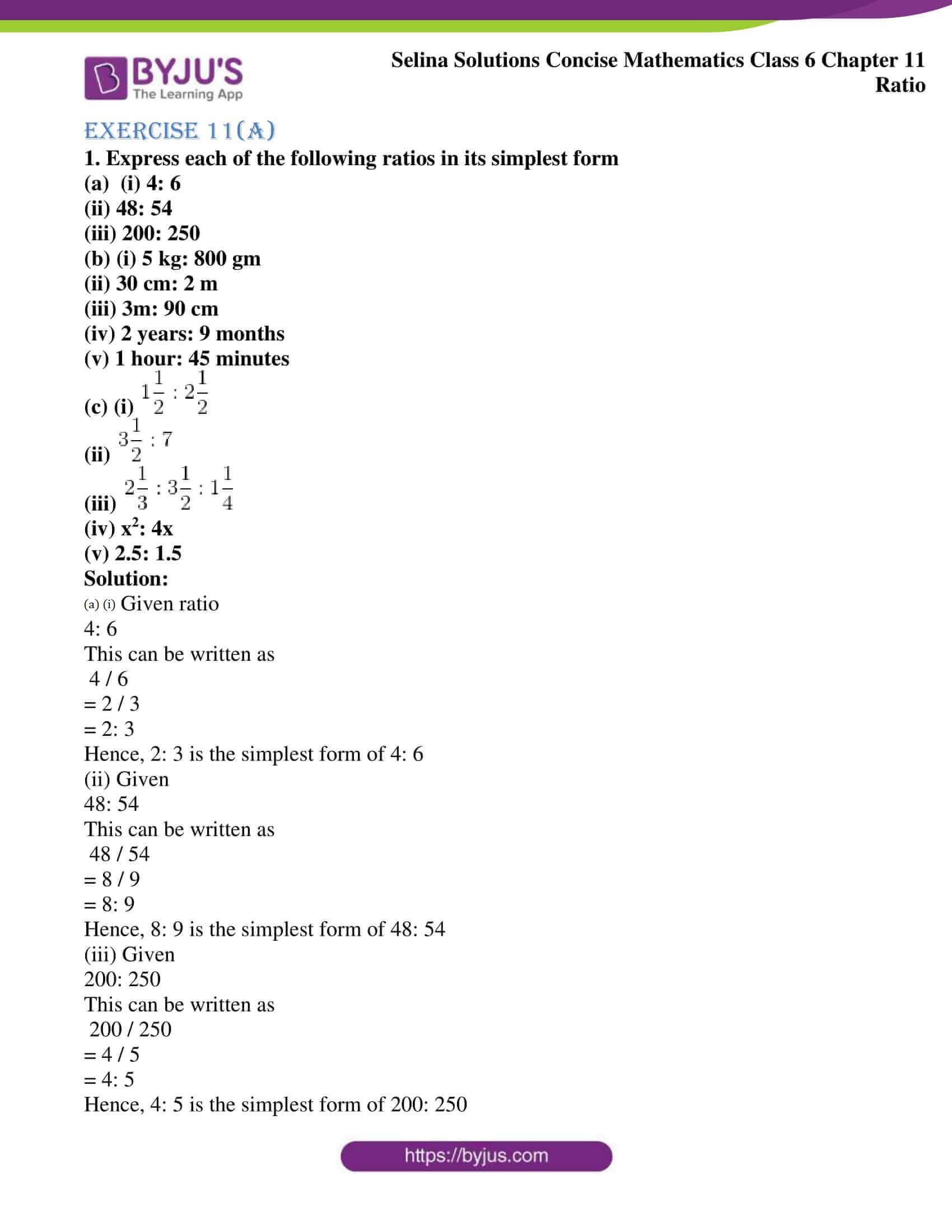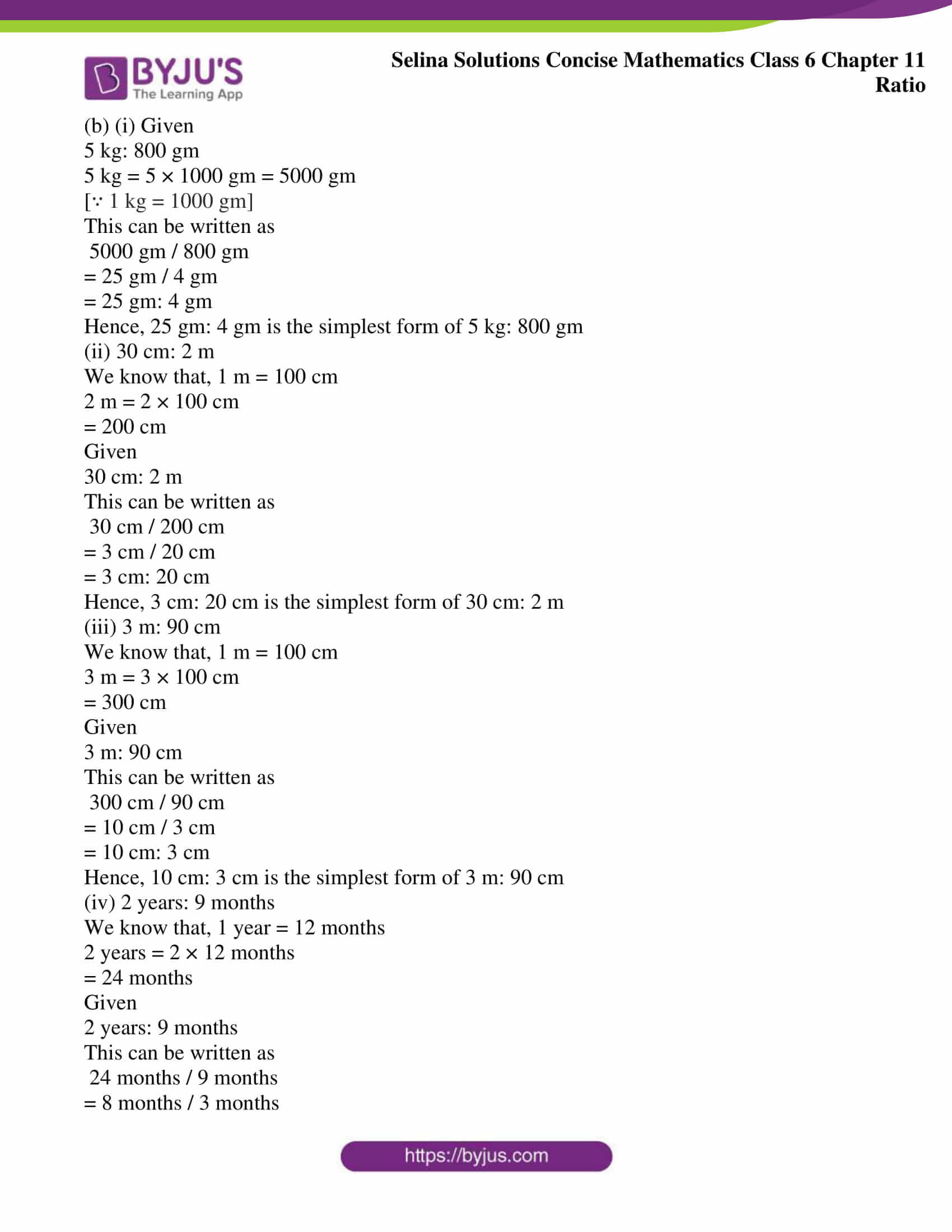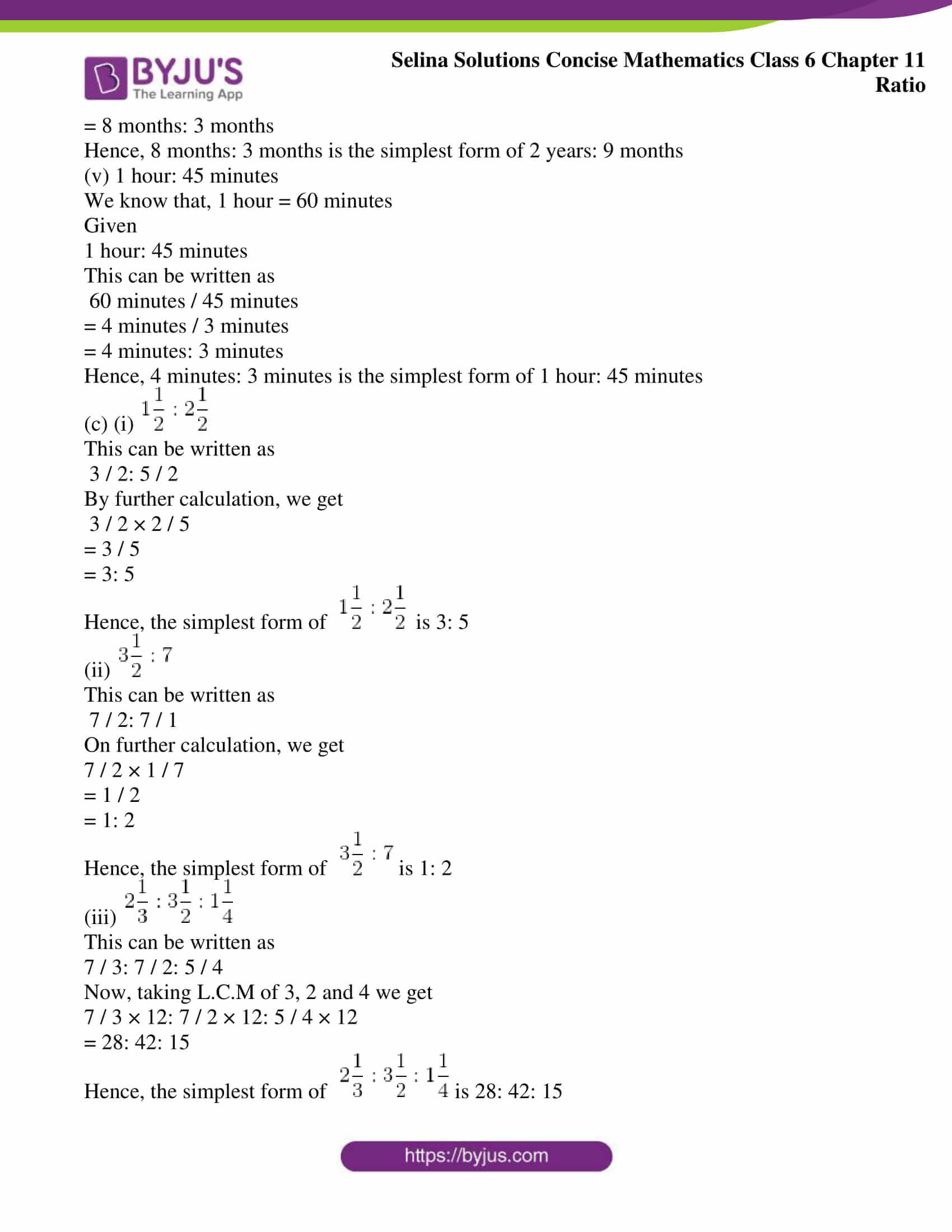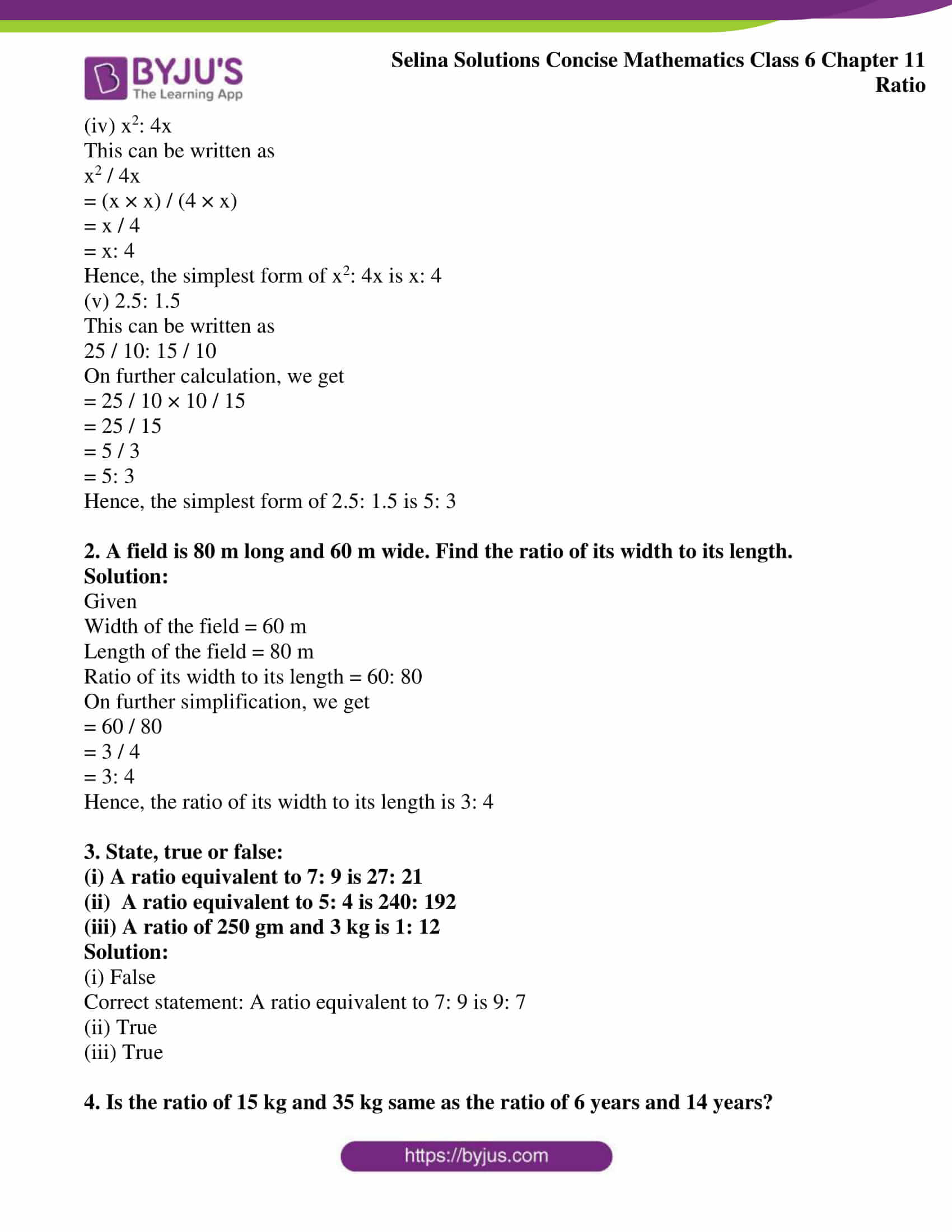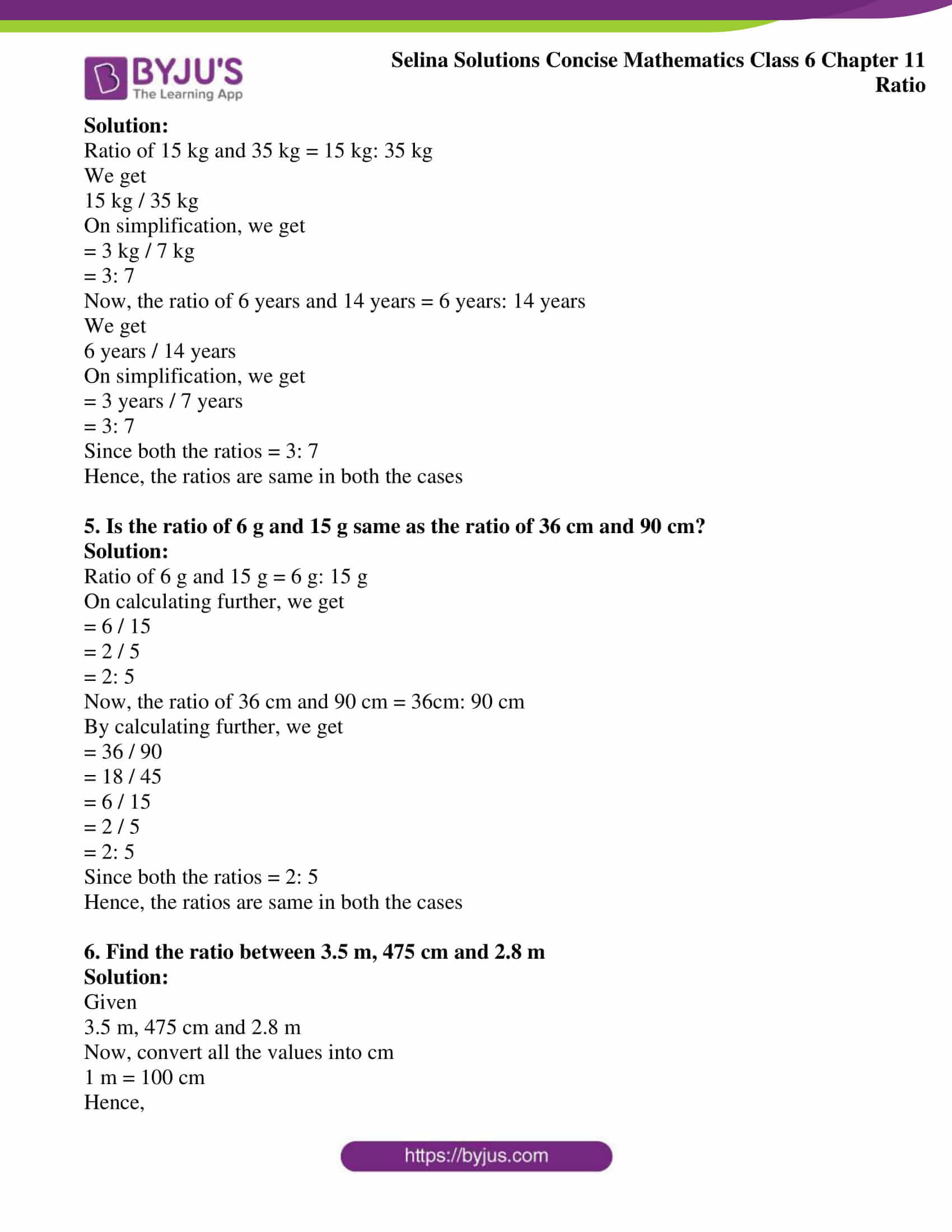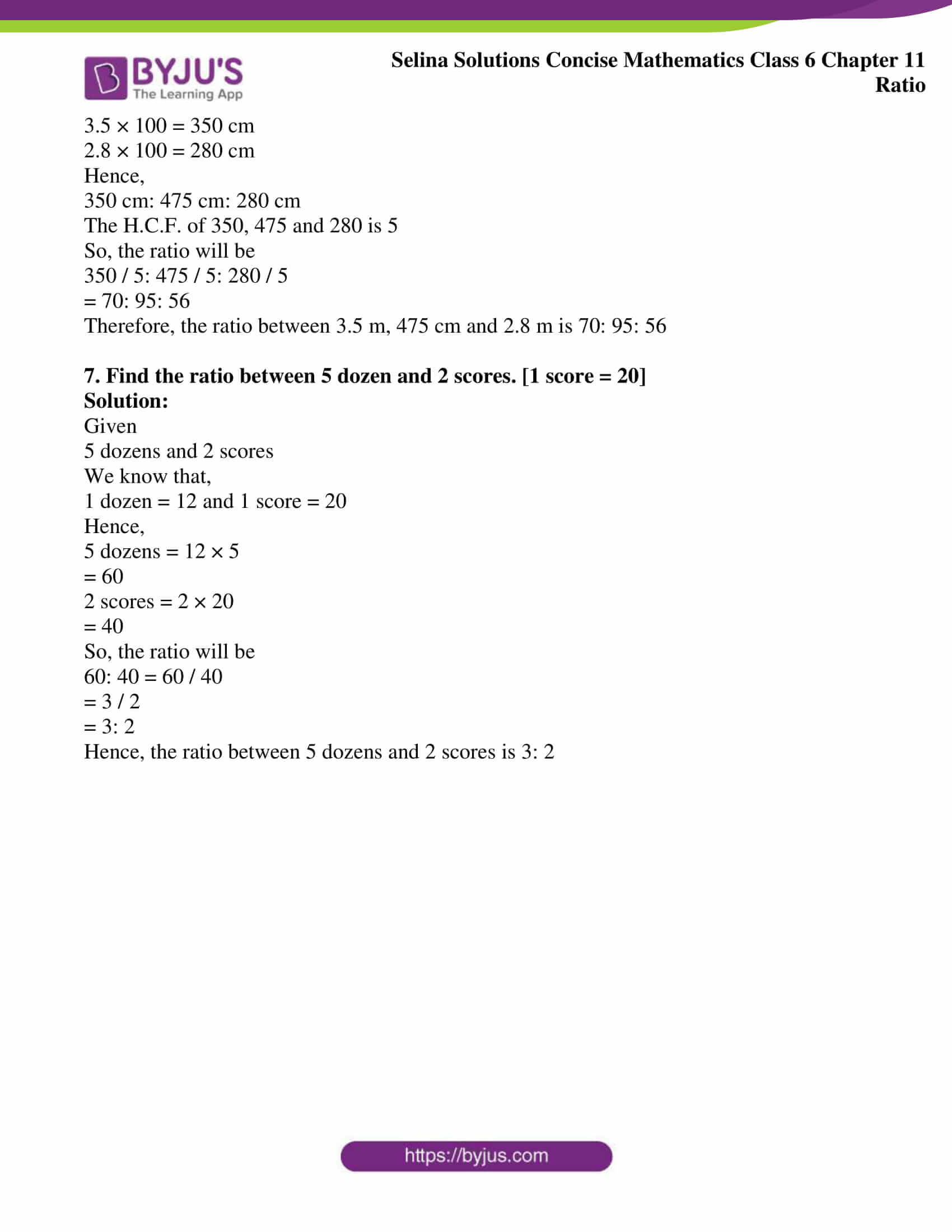### Access other exercises of Selina Solutions Concise Mathematics Class 6 Chapter 11: Ratio

Exercise 11(B) Solutions

Exercise 11(C) Solutions

Exercise 11 (D) Solutions

### Access Selina Solutions Concise Mathematics Class 6 Chapter 11: Ratio Exercise 11(A)

#### Exercise 11(A)

1. Express each of the following ratios in its simplest form

(a) (i) 4: 6

(ii) 48: 54

(iii) 200: 250

(b) (i) 5 kg: 800 gm

(ii) 30 cm: 2 m

(iii) 3m: 90 cm

(iv) 2 years: 9 months

(v) 1 hour: 45 minutes

(c) (i)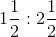(ii)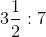(iii)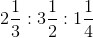(iv) x2: 4x

(v) 2.5: 1.5

Solution:

(a) (i) Given ratio

4: 6

This can be written as

4 / 6

= 2 / 3

= 2: 3

Hence, 2: 3 is the simplest form of 4: 6

(ii) Given

48: 54

This can be written as

48 / 54

= 8 / 9

= 8: 9

Hence, 8: 9 is the simplest form of 48: 54

(iii) Given

200: 250

This can be written as

200 / 250

= 4 / 5

= 4: 5

Hence, 4: 5 is the simplest form of 200: 250

(b) (i) Given

5 kg: 800 gm

5 kg = 5 × 1000 gm = 5000 gm

[∵ 1 kg = 1000 gm]

This can be written as

5000 gm / 800 gm

= 25 gm / 4 gm

= 25 gm: 4 gm

Hence, 25 gm: 4 gm is the simplest form of 5 kg: 800 gm

(ii) 30 cm: 2 m

We know that, 1 m = 100 cm

2 m = 2 × 100 cm

= 200 cm

Given

30 cm: 2 m

This can be written as

30 cm / 200 cm

= 3 cm / 20 cm

= 3 cm: 20 cm

Hence, 3 cm: 20 cm is the simplest form of 30 cm: 2 m

(iii) 3 m: 90 cm

We know that, 1 m = 100 cm

3 m = 3 × 100 cm

= 300 cm

Given

3 m: 90 cm

This can be written as

300 cm / 90 cm

= 10 cm / 3 cm

= 10 cm: 3 cm

Hence, 10 cm: 3 cm is the simplest form of 3 m: 90 cm

(iv) 2 years: 9 months

We know that, 1 year = 12 months

2 years = 2 × 12 months

= 24 months

Given

2 years: 9 months

This can be written as

24 months / 9 months

= 8 months / 3 months

= 8 months: 3 months

Hence, 8 months: 3 months is the simplest form of 2 years: 9 months

(v) 1 hour: 45 minutes

We know that, 1 hour = 60 minutes

Given

1 hour: 45 minutes

This can be written as

60 minutes / 45 minutes

= 4 minutes / 3 minutes

= 4 minutes: 3 minutes

Hence, 4 minutes: 3 minutes is the simplest form of 1 hour: 45 minutes

(c) (i)This can be written as

3 / 2: 5 / 2

By further calculation, we get

3 / 2 × 2 / 5

= 3 / 5

= 3: 5

Hence, the simplest form ofis 3: 5

(ii)This can be written as

7 / 2: 7 / 1

On further calculation, we get

7 / 2 × 1 / 7

= 1 / 2

= 1: 2

Hence, the simplest form ofis 1: 2

(iii)This can be written as

7 / 3: 7 / 2: 5 / 4

Now, taking L.C.M of 3, 2 and 4 we get

7 / 3 × 12: 7 / 2 × 12: 5 / 4 × 12

= 28: 42: 15

Hence, the simplest form ofis 28: 42: 15

(iv) x2: 4x

This can be written as

x2 / 4x

= (x × x) / (4 × x)

= x / 4

= x: 4

Hence, the simplest form of x2: 4x is x: 4

(v) 2.5: 1.5

This can be written as

25 / 10: 15 / 10

On further calculation, we get

= 25 / 10 × 10 / 15

= 25 / 15

= 5 / 3

= 5: 3

Hence, the simplest form of 2.5: 1.5 is 5: 3

2. A field is 80 m long and 60 m wide. Find the ratio of its width to its length.

Solution:

Given

Width of the field = 60 m

Length of the field = 80 m

Ratio of its width to its length = 60: 80

On further simplification, we get

= 60 / 80

= 3 / 4

= 3: 4

Hence, the ratio of its width to its length is 3: 4

3. State, true or false:

(i) A ratio equivalent to 7: 9 is 27: 21

(ii) A ratio equivalent to 5: 4 is 240: 192

(iii) A ratio of 250 gm and 3 kg is 1: 12

Solution:

(i) False

Correct statement: A ratio equivalent to 7: 9 is 9: 7

(ii) True

(iii) True

4. Is the ratio of 15 kg and 35 kg same as the ratio of 6 years and 14 years?

Solution:

Ratio of 15 kg and 35 kg = 15 kg: 35 kg

We get

15 kg / 35 kg

On simplification, we get

= 3 kg / 7 kg

= 3: 7

Now, the ratio of 6 years and 14 years = 6 years: 14 years

We get

6 years / 14 years

On simplification, we get

= 3 years / 7 years

= 3: 7

Since both the ratios = 3: 7

Hence, the ratios are same in both the cases

5. Is the ratio of 6 g and 15 g same as the ratio of 36 cm and 90 cm?

Solution:

Ratio of 6 g and 15 g = 6 g: 15 g

On calculating further, we get

= 6 / 15

= 2 / 5

= 2: 5

Now, the ratio of 36 cm and 90 cm = 36cm: 90 cm

By calculating further, we get

= 36 / 90

= 18 / 45

= 6 / 15

= 2 / 5

= 2: 5

Since both the ratios = 2: 5

Hence, the ratios are same in both the cases

6. Find the ratio between 3.5 m, 475 cm and 2.8 m

Solution:

Given

3.5 m, 475 cm and 2.8 m

Now, convert all the values into cm

1 m = 100 cm

Hence,

3.5 × 100 = 350 cm

2.8 × 100 = 280 cm

Hence,

350 cm: 475 cm: 280 cm

The H.C.F. of 350, 475 and 280 is 5

So, the ratio will be

350 / 5: 475 / 5: 280 / 5

= 70: 95: 56

Therefore, the ratio between 3.5 m, 475 cm and 2.8 m is 70: 95: 56

7. Find the ratio between 5 dozen and 2 scores. [1 score = 20]

Solution:

Given

5 dozens and 2 scores

We know that,

1 dozen = 12 and 1 score = 20

Hence,

5 dozens = 12 × 5

= 60

2 scores = 2 × 20

= 40

So, the ratio will be

60: 40 = 60 / 40

= 3 / 2

= 3: 2

Hence, the ratio between 5 dozens and 2 scores is 3: 2